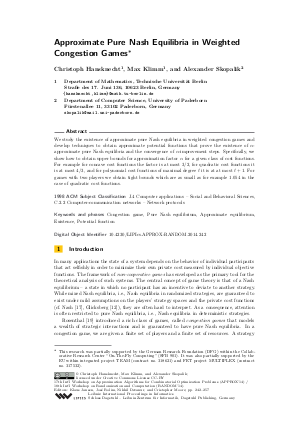Document# Approximate Pure Nash Equilibria in Weighted Congestion Games

### Authors Christoph Hansknecht, Max Klimm, Alexander Skopalik## File

LIPIcs.APPROX-RANDOM.2014.242.pdf
• Filesize: 0.48 MB
• 16 pages

## Cite As

Christoph Hansknecht, Max Klimm, and Alexander Skopalik. Approximate Pure Nash Equilibria in Weighted Congestion Games. In Approximation, Randomization, and Combinatorial Optimization. Algorithms and Techniques (APPROX/RANDOM 2014). Leibniz International Proceedings in Informatics (LIPIcs), Volume 28, pp. 242-257, Schloss Dagstuhl - Leibniz-Zentrum für Informatik (2014)
https://doi.org/10.4230/LIPIcs.APPROX-RANDOM.2014.242

## Abstract

We study the existence of approximate pure Nash equilibria in weighted congestion games and develop techniques to obtain approximate potential functions that prove the existence of alpha-approximate pure Nash equilibria and the convergence of alpha-improvement steps. Specifically, we show how to obtain upper bounds for approximation factor alpha for a given class of cost functions. For example for concave cost functions the factor is at most 3/2, for quadratic cost functions it is at most 4/3, and for polynomial cost functions of maximal degree d it is at at most d + 1. For games with two players we obtain tight bounds which are as small as for example 1.054 in the case of quadratic cost functions.
##### Keywords
• Congestion game
• Pure Nash equilibrium
• Approximate equilibrium
• Existence
• Potential function

## Metrics

• Access Statistics
• Total Accesses (updated on a weekly basis)
0